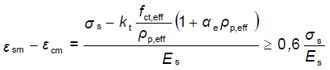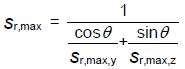US: Tel: 1-206-279-3300
EU: Tel: +30 6986 007 252
• FREE VERSION OF RCsolver - Concrete Design with EC2, EC8, and ACI 318

Get full capabiltiies with our free version of RC-Solver once you signup for our newsletter. With the free version you will have to wait 30 seconds for advertisements.

# CRACK WIDTH CALCULATIONS ACCORDING TO EUROCODE 2

Eurocode 2 (EN1992-1-1) proposes equations for the calculation of crack width, taking into account several parameters, like the concrete and steel strain and reinforcing bar diameters.

According to Eurocode 2, the crack width, wk, can be calculated from the following equation:

wk = sr,max (εsm –εcm)

, where

sr,max:            the maximum crack spacing

εsm:                 the mean strain in the reinforcement under the relevant combination of loads, including the effect of imposed deformations and taking into account the effects of tension stiffening. Only the additional tensile strain beyond the state of zero strain of the concrete at the same level is considered

εcm:                the mean strain in the concrete between cracks

• The difference εsm –εcm can be calculated from the following expression:, where:

σs:                   the stress in the tension reinforcement assuming a cracked section. For pretensioned members, σs may be replaced by Δσp the stress variation in prestressing tendons from the state of zero strain of the concrete at the same level.

Αe:                   the ratio Es/Ecm

ρp,eff               (As + ξ12 Ap’)/Ac,eff ,where

Ap‘ is the area of pre or post-tensioned tendons within Ac,eff.

Ac,eff is the effective area of concrete in tension surrounding the reinforcement or prestressing tendons of depth, hc,ef , where hc,ef is the lesser of  2,5(h-d), (h-x)/3 or h/2, where

ξ: ratio of bond strength of prestressing and reinforcing steel

φs: largest bar diameter of reinforcing steel

φp: equivalent diameter of tendon

kt:                    a factor dependent on the duration of the load

• In situations where bonded reinforcement is fixed at reasonably close centers within the tension zone (spacing ≤ 5(c+φ/2), the maximum final crack spacing should be calculated from the following equation:

sr,max = k3c + k1k2k4φ /ρp,eff

, where:

φ:                     he bar diameter. Where a mixture of bar diameters is used in a section, an equivalent diameter, φeq, should be used. For a section with n1 bars of diameter φ1 and n2 bars of diameter φ2, we should use the following equation:c:                     the cover to the longitudinal reinforcement

k1:                   a coefficient which takes account of the bond properties of the bonded reinforcement:

= 0,8 for high bond bars

= 1,6 for bars with an effectively plain surface (e.g. prestressing tendons)

k2:                   a coefficient which takes account of the distribution of strain:

= 0,5 for bending

= 1,0 for pure tension

For cases of eccentric tension or for local areas, intermediate values of k2 should be used which may be calculated from the equation:

k2 = (ε1 + ε2)/2ε1 (7.13) , where

ε1 is the greater and ε2 is the lesser tensile strain at the boundaries of the section considered, assessed on the basis of a cracked section

Note: The values of k3 and k4 for use in a Country may be found in its National Annex. The recommended values are 3,4 and 0,425 respectively.

Where the spacing of the bonded reinforcement exceeds 5(c+φ/2) or where there is no bonded reinforcement within the tension zone, an upper bound to the crack width should be calculated by assuming a maximum crack spacing:

sr,max = 1,3 (h - x)

Where the angle between the axes of principal stress and the direction of the reinforcement, for members reinforced in two orthogonal directions, is significant (>15°), then the crack spacing sr,max may be calculated from the following equation:, where:

θ:                                 the angle between the reinforcement in the y direction and the direction of the principal tensile stress

sr,max,y, sr,max,z:      the crack spacings calculated in the y and z directions respectively

• For walls subjected to early thermal contraction where the horizontal steel area, As does not fulfil minimum reinforcement requirements and where the bottom of the wall is restrained by a previously cast base, sr,max may be assumed to be equal to 1.3 times the height of the wall.

# E-mail List Signup

Signup to our Email List for the latest information about our products, support and more.